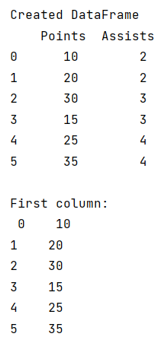# How to get the first column of a pandas DataFrame as a Series?

Given a Pandas DataFrame, we have to get the first column of a pandas DataFrame as a Series. By Pranit Sharma Last updated : September 22, 2023

Pandas is a special tool which allows us to perform complex manipulations of data effectively and efficiently. Inside pandas, we mostly deal with a dataset in the form of DataFrame.

Columns are the different fields which contains their particular values when we create a DataFrame. We can perform certain operations on both rows & column values.

## Problem statement

Given a Pandas DataFrame, we have to get the first column of a pandas DataFrame as a Series.

## Getting the first column of a pandas DataFrame as a Series

To get the first column of pandas DataFrame as a series, we will use pandas.DataFrame.iloc property. The iloc[] property gets, or sets, the value(s) of the specified indexes.

Note

To work with pandas, we need to import pandas package first, below is the syntax:

```import pandas as pd
```

Let us understand with the help of an example,

## Python program to get the first column of a pandas DataFrame as a Series

```# Importing pandas package
import pandas as pd

# Creating a dictionary
d= {
'Points':[10,20,30,15,25,35],
'Assists':[2,2,3,3,4,4]
}

# Creating a DataFrame
df = pd.DataFrame(d)

# Display DataFrame
print("Created DataFrame\n",df,"\n")

# Get First column
first_col = df.iloc[:, 0]

# Display First column
print("First column:\n",first_col)
```

### Output

The output of the above program is: